# Continuity and Differentiability class 12 Notes Mathematics

Continuity and Differentiability Class 12 Notes Mathematics in PDF are available for free download in myCBSEguide mobile app. The best app for CBSE students now provides Continuity and Differentiability class 12 Notes latest chapter wise notes for quick preparation of CBSE board exams and school-based annual examinations. Class 12 Mathematics notes on chapter 5 Continuity and Differentiability are also available for download in CBSE Guide website.

## Continuity and Differentiability Class 12 Notes Mathematics

Download CBSE class 12th revision notes for chapter 5 Continuity and Differentiability in PDF format for free. Download revision notes for Continuity and Differentiability class 12 Notes and score high in exams. These are the Continuity and Differentiability class 12 Notes prepared by team of expert teachers. The revision notes help you revise the whole chapter 5 in minutes. Revision notes in exam days is one of the best tips recommended by teachers during exam days.

## CBSE Class 12 Mathematics Chapter 5 Continuity and Differentiability

• Continuity of function at a point: Geometrically we say that a function  is continuous at  if the graph of the function  is continuous (without any break) at .
• A funciton  is said to be continuous at a point  if:

(i)      exists i.e.,  is finite, definite and real.

(ii)     exists.

(iii)

• A function  is continuous at   if  where  through positive values.
• Continuity of a function in a closed interval: A function  is said to be continuous in the closed interval  if it is continuous for every value of  lying between a and b continuous to the right of a and to the left of  i.e.,  and
• Continuity of a function in a open interval: A function  is said to be continuous in an open interval  if it is continuous at every point in  .
• Discontinuity (Discontinuous function): A function  is said to be discontinuous in an interval if it is discontinuous even at a single point of the interval.
• Suppose  is a real function and  is a point in its domain. The derivative of  at  is defined by  provided this limit exists.
• A real valued function is continuous at a point in its domain if the limit of the function at that point equals the value of the function at that point. A function is continuous if it is continuous on the whole of its domain.
•  is derivative of first order and is also denoted by  or .
• Sum, difference, product and quotient of continuous functions are continuous. i.e., if f and g are continuous functions, then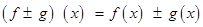is continuous. (f . g) (x) = f (x) . g(x) is continuous.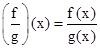(wherever g (x) ≠ 0) is continuous.

• Every differentiable function is continuous, but the converse is not true.
• Chain rule is rule to differentiate composites of functions. If f = v o u, t = u (x) and if both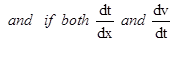exist then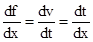• Following are some of the standard derivatives (in appropriate domains):
•  [Product Rule]
• , wherever  [Quotient Rule]
• If  then  [Chain Rule]
• If , then  [Parametric Form]
•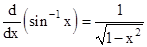•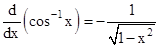•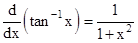•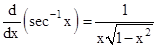•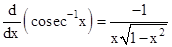•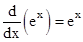• Logarithmic differentiation is a powerful technique to differentiate functions of the form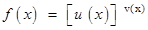Here both f (x) and u (x) need to be positive for this technique to make sense.
• If we have to differentiate logarithmic funcitons, other than base , then we use the result  and then differentiate R.H.S.
• While differentiating inverse trigonometric functions, first represent it in simplest form by using suitable substitution and then differentiate simplified form.
• If we are given implicit functions then differentiate directly w.r.t. suitable variable involved and get the derivative by readusting the terms.
•  is derivative of second order and is denoted by  or .
• Rolle’s Theorem: If f: [a, b] → R is continuous on [a, b] and differentiable on (a, b) such that f (a) = f (b), then there exists some c in (a, b) such that f ′(c) = 0.
• Lagrange’s Mean Value Theorem: If f: [a, b] → R is continuous on [a, b] and differentiable on (a, b). Then there exists some c in (a, b) such that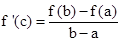## CBSE Class 12 Revision Notes and Key Points

Continuity and Differentiability class 12 Notes Mathematics. CBSE quick revision note for class-12 Chemistry Physics Math’s, Biology and other subject are very helpful to revise the whole syllabus during exam days. The revision notes covers all important formulas and concepts given in the chapter. Even if you wish to have an overview of a chapter, quick revision notes are here to do if for you. These notes will certainly save your time during stressful exam days.

To download Continuity and Differentiability class 12 Notes, sample paper for class 12 Physics, Chemistry, Biology, History, Political Science, Economics, Geography, Computer Science, Home Science, Accountancy, Business Studies and Home Science; do check myCBSEguide app or website. myCBSEguide provides sample papers with solution, test papers for chapter-wise pract, ce, NCERT Continuity and Differentiability, NCERT Exemplar Continuity and Differentiability, quick revision notes for ready reference, CBSE guess papers and CBSE important question papers. Sample Paper all are made available through the best app for CBSE students and myCBSEguide website.### Test Generator

Create question paper PDF and online tests with your own name & logo in minutes.### myCBSEguide

Question Bank, Mock Tests, Exam Papers, NCERT Solutions, Sample Papers, Notes